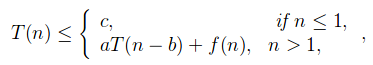# Master Theorem For Subtract and Conquer Recurrences

• Difficulty Level : Medium
• Last Updated : 31 May, 2021

Master theorem is used to determine the Big – O upper bound on functions which possess recurrence, i.e which can be broken into sub problems.
Master Theorem For Subtract and Conquer Recurrences
Let T(n) be a function defined on positive n as shown below:for some constants c, a>0, b>0, k>=0 and function f(n). If f(n) is O(nk), then
1. If a<1 then T(n) = O(nk
2. If a=1 then T(n) = O(nk+1
3. if a>1 then T(n) = O(nkan/b)
Proof of above theorem( By substitution method ):
From above function, we have:
T(n) = aT(n-b) + f(n)
T(n-b) = aT(n-2b) + f(n-b)
T(n-2b) = aT(n-3b) + f(n-2b)
Now,
T(n-b) = a2T(n-3b) + af(n-2b) + f(n-b)
T(n) = a3T(n-3b) + a2f(n-2b) + af(n-b) + f(n)
T(n) = Σi=0 to n ai f(n-ib) + constant, where f(n-ib) is O(n-ib)
T(n) = O(nk Σi=0 to n/b ai )

Where,
If a<1 then Σi=0 to n/b ai = O(1), T(n) = O(nk)
If a=1 then Σi=0 to n/b ai = O(n), T(n) = O(nk+1
If a>1 then Σi=0 to n/b ai = O(an/b), T(n) = O(nkan/b)
Consider the following program for nth fibonacci number

## C++

 `#include``int` `fib(``int` `n)``{``   ``if` `(n <= 1)``      ``return` `n;``   ``return` `fib(n-1) + fib(n-2);``}`` ` `int` `main ()``{``  ``int` `n = 9;``  ``printf``(``"%d"``, fib(n));``  ``getchar``();``  ``return` `0;``}`

## Python3

 `# Python3 code for the above approach``def` `fib(n):``    ``if` `(n <``=` `1``):``        ``return` `n``    ``return` `fib(n ``-` `1``) ``+` `fib(n ``-` `2``)` `# Driver code``n ``=` `9``print``(fib(n))` `# This code is contributed``# by sahishelangia`

## Java

 `//Java code for above the approach.``class` `clg``{`` ``static` `int` `fib(``int` `n)``{``if` `(n <= ``1``)``    ``return` `n;``return` `fib(n-``1``) + fib(n-``2``);``}``// Driver Code``public` `static` `void` `main (String[] args)``{``int` `n = ``9``;``System.out.println( fib(n));``}``}``// This code is contributed by Mukul Singh.`

## C#

 `// C# code for above the approach.``using` `System;``    ` `class` `GFG``{``    ``static` `int` `fib(``int` `n)``    ``{``        ``if` `(n <= 1)``            ``return` `n;``        ``return` `fib(n - 1) + fib(n - 2);``    ``}``    ` `    ``// Driver Code``    ``public` `static` `void` `Main(String[] args)``    ``{``        ``int` `n = 9;``        ``Console.WriteLine(fib(n));``    ``}``}` `// This code has been contributed``// by Rajput-Ji`

## PHP

 ``

## Javascript

 ``

Output

`34`

Time complexity Analysis:
The recursive function can be defined as, T(n) = T(n-1) + T(n-2)

• For Worst Case, Let T(n-1) ≈ T(n-2)
T(n) = 2T(n-1) + c
where,f(n) = O(1)
∴ k=0, a=2, b=1;
T(n) = O(n02n/1
= O(2n

• For Best Case, Let T(n-2) ≈ T(n-1)
T(n) = 2T(n-2) + c
where,f(n) = O(1)
∴ k=0, a=2, b=2;
T(n) = O(n02n/2
= O(2n/2

More Examples:

• Example-1
T(n) = 3T(n-1), n>0
= c, n<=0
Sol:a=3, b=1, f(n)=0 so k=0;
Since a>0, T(n) = O(nkan/b
T(n)= O(n03n/1
T(n)= 3n

• Example-2
T(n) = T(n-1) + n(n-1), if n>=2
= 1, if n=1
Sol:a=1, b=1, f(n)=n(n-1) so k=2;
Since a=1, T(n) = O(nk+1
T(n)= O(n2+1
T(n)= O(n3

• Example-3
T(n) = 2T(n-1) – 1, if n>0
= 1, if n<=0
Sol: This recurrence can’t be solved using above method
since function is not of form T(n) = aT(n-b) + f(n)

This article is contributed by Yash Singla. If you like GeeksforGeeks and would like to contribute, you can also write an article using write.geeksforgeeks.org or mail your article to contribute@geeksforgeeks.org. See your article appearing on the GeeksforGeeks main page and help other Geeks.